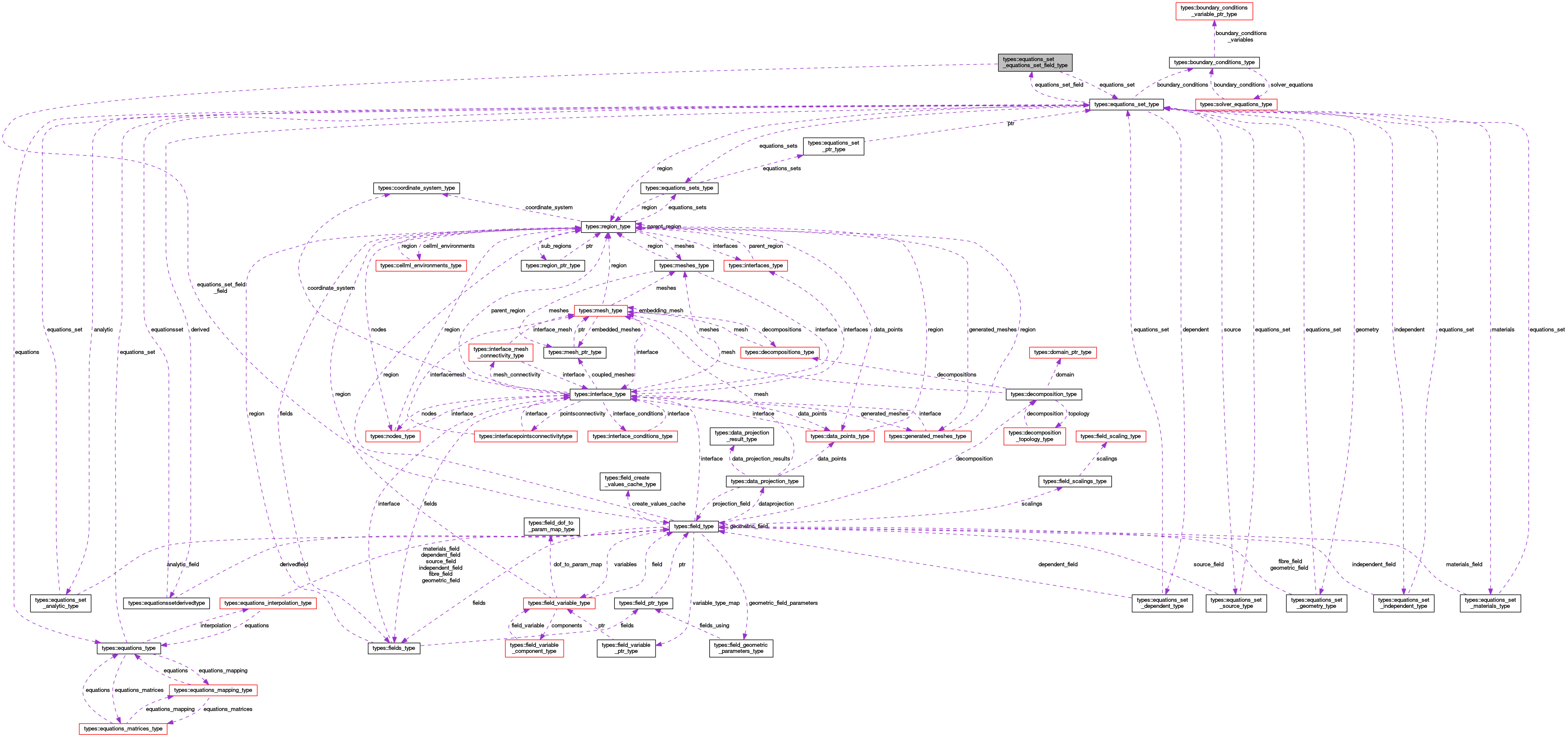OpenCMISS-Iron Internal API Documentation
types::equations_set_equations_set_field_type Type Reference
Collaboration diagram for types::equations_set_equations_set_field_type:[legend]

## Public Attributes

type(equations_set_type), pointer equations_set
A pointer to the equations set. More...

logical equations_set_field_finished
Is .TRUE. if the equations set field for the equations set has finished being created, .FALSE. if not. More...

logical equations_set_field_auto_created
Is .TRUE. if the equations set field has been auto created, .FALSE. if not. More...

type(field_type), pointer equations_set_field_field
A pointer to the equations set field for the equations set. More...

## Detailed Description

Definition at line 1933 of file types.f90.

## Member Data Documentation

 type(equations_set_type), pointer types::equations_set_equations_set_field_type::equations_set

A pointer to the equations set.

Definition at line 1934 of file types.f90.

 logical types::equations_set_equations_set_field_type::equations_set_field_auto_created

Is .TRUE. if the equations set field has been auto created, .FALSE. if not.

Definition at line 1936 of file types.f90.

 type(field_type), pointer types::equations_set_equations_set_field_type::equations_set_field_field

A pointer to the equations set field for the equations set.

Definition at line 1937 of file types.f90.

 logical types::equations_set_equations_set_field_type::equations_set_field_finished

Is .TRUE. if the equations set field for the equations set has finished being created, .FALSE. if not.

Definition at line 1935 of file types.f90.